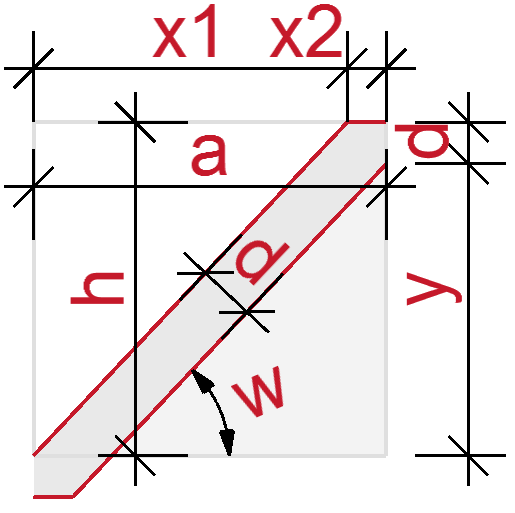# ProblemThe following I call handrail problem, because I needed last time, when I constructed a handrail. I ran into this the second time and spent too much time in thinking about. Given is...with fixed parameters for "a", "d" and "h". I am looking for the right coordinates of the polygon, finally for "w".

# SolutionThere is no good solution so far. Just an iteration loop you find below.

The formulas are:

TAN(w)   = h/x1

SIN(w/2) = x2/d

a = x1+x2

h = y+d

But no way (for me) to solve for w.

# Script!»» iteration

ww=ATN(h/a)

FOR i=1 TO 15
x1=h/TAN(ww)
x2=d*TAN(ww/2)
x=(x1+x2)-a
ww=ATN(h/(a-x2+x/2))
NEXT i

Diese Website benutzt Cookies um die Nutzererfahrung zu verbessern. Datenschutzerklärung